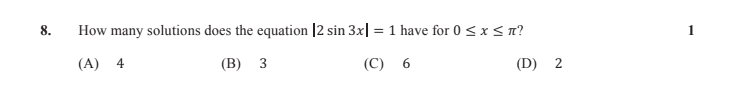# Solving absolute values of trigonometry

## Homework Statement## The Attempt at a Solution

2sin3x=1 OR 2sin3x= -1
sin3x=1/2 sin3x= -1/2
From the unit circle and in accordance with the domain
there are 3 solutions (B)
HOW?

Ray Vickson
Homework Helper
Dearly Missed

## Homework Statement

View attachment 229661

## The Attempt at a Solution

2sin3x=1 OR 2sin3x= -1
sin3x=1/2 sin3x= -1/2
From the unit circle and in accordance with the domain
there are 3 solutions (B)